## Convergent Series

The infinite Series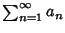is convergent if the Sequence of partial sums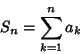is convergent. Conversely, a Series is divergent if the Sequence of partial sums is divergent. If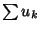andare convergent Series, thenand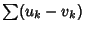are convergent. If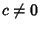, thenand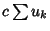both converge or both diverge. Convergence and divergence are unaffected by deleting a finite number of terms from the beginning of a series. Constant terms in the denominator of a sequence can usually be deleted without affecting convergence. All but the highest Power terms in Polynomials can usually be deleted in both Numerator and Denominator of a Series without affecting convergence. If a Series converges absolutely, then it converges.